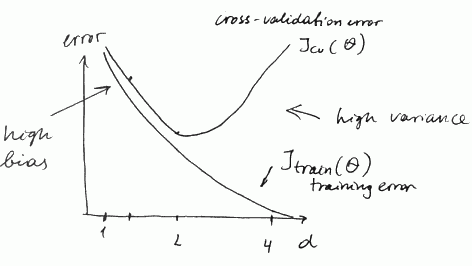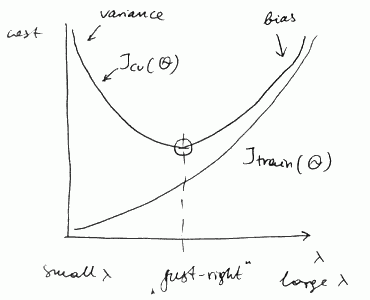# ML Wiki

## Machine Learning Diagnosis

Suppose you created a model, but when you tested it, you found that it makes large errors

What should you try?

• Get more training examples
• Try smaller set of features
• Try adding polynomial features (beware of Overfitting!)
• Try increasing regularization parameter $\lambda$
• Try decreasing $\lambda$

Diagnosis - a test that you can run to gain insights what is working with the learning algorithms and what is not, and gain guidance as how to improve the performance.

### Evaluating a Hypothesis

To test if we overfit, we can perform Cross-Validation:

• train the model on the training set
• check the model on the test set

## Diagnosing Bias vs Variance

the main sources of problems are

### Fitting Polynomial

How to distinguish between them and say which one of them we experience?

• Suppose we want to fit parameter $d$ - what degree of polynomial to use (see here)
• with $d = 1$ we underfit
• with $d = 2$ we are just right
• with $d = 4$ we overfit

We can plot the cost function errors vs degree of polynomial $d$ for

• the training set $J_{\text{train}}(\theta)$
• the cross-validation (or test) set $J_{\text{cv}}(\theta)$in case of bias (underfit) we have

• both $J_{\text{train}}(\theta)$ and $J_{\text{cv}}(\theta)$ are high
• and $J_{\text{train}}(\theta) \approx J_{\text{cv}}(\theta)$

in case of variance (overfit)

• $J_{\text{train}}(\theta)$ is low, $but J_{\text{cv}}(\theta)$ is high
• and $J_{\text{cv}}(\theta) \gg J_{\text{train}}(\theta)$ (much greater)

### Fitting Regularization Parameter

When we try to find the best Regularization parameter for a hypothesis we get similar curves:• with small $\lambda$ we have high variance
• with large $\lambda$ we have high bias

### Learning Curves

Learning Curves is a technique that is used to

## What To Do Next?

So, depending on what kind of problem we have, we should decide what to do next

To fix high variance:

To fix high bias: